# NCERT Solutions Class 12 Chemistry Chapter 3 ELECTROCHEMISTRY

Ncert Solutions for class 12 subject Chemistry Chapter 3 ELECTROCHEMISTRYin pdf Best Free NCERT Solutions for class 1 to 12 in pdf NCERT Solutions, cbse board, Chemistry, ncert Solutions for Class 12 Chemistry, class 12 Chemistry ncert solutions, ELECTROCHEMISTRY, Class 12, ncert solutions chapter 3 ELECTROCHEMISTRY, class 12 Chemistry, class 12 Chemistry ncert solutions, Chemistry ncert solutions class 12, Ncert Solutions Class 12 Chemistry Chapter 3 ELECTROCHEMISTRY

## Concept Chapter 3 Class 12 Chemistry ELECTROCHEMISTRY Ncert Solutions

Q-1What is electrochemistry?
Electrochemistry is the study of production of electricity from energy released during spontaneous chemical reactions.

Q-2 What are the uses of electrochemistry?
1. bring about non-spontaneous chemical transformations.
2. A large number of metals, sodium hydroxide, chlorine, fluorine and many other chemicals are produced by electrochemical methods.
3. Electrochemistry is important for creating new technologies that are ecofriendly.
4. The transmission of sensory signals through cells to brain and vice versa and communication between the cells are known to have electrochemical origin.
5. Electrochemical cells are extensively used for determining the pH of solutions, solubility product, equilibrium constant and other thermodynamic properties and for potentiometric titrations.

Q-3 What are the construction and functioning of Daniell cell or galvanic cell .
This cell converts the chemical energy liberated during the redox reaction in to electrical energy and has an electrical potential equal to 1.1 V when concentration of Zn2+ and Cu2+ ions is unity (1 molar)
< style="margin-left:35px;">Zn(s) + Cu2+(aq) → Zn2+ (aq) + Cu(s) This reaction is a combination of two half reactions whose addition gives the overall cell reaction
The reduction half reaction occurs on the copper electrode so that copper electrode may be called the reduction half cell
< style="margin-left:35px;">Cu2+ + 2e → Cu(s) Oxidation half reaction occurs on the zinc electrode so that zinc electrode may be called the oxidation half cell
< style="margin-left:35px;">Zn(s) → Zn2+ + 2e The electrolytes of the two half-cells are connected internally through a salt bridge as shown in Fig. 3.1. Sometimes, both the electrodes dip in the same electrolyte solution and in such cases we don’t require a salt bridge.
In this device the Gibbs energy of the spontaneous redox reaction is converted into electrical work which may be used for running a motor or other electrical gadgets like heater, fan, geyser, etc.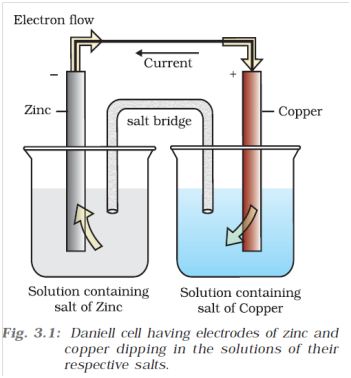Case-1 when Ecell > External opposite potential

If an external opposite potential is applied [Fig. 3.2(a)] and increased slowly, we find that the reaction continues to take place till the opposing voltage reaches the value 1.1 V [Fig. 3.2(b)]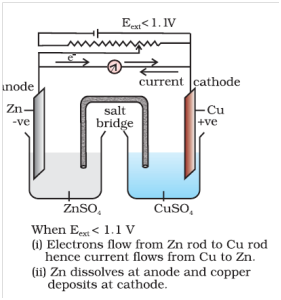Case-2 when Ecell = External opposite potential
When opposing voltage reaches at the value 1.1 V [Fig. 3.2(b)], the reaction stops and no further current flows through the cell takes place.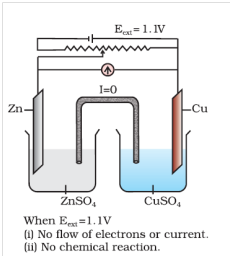Case- 3 when Ecell < External opposite potential
When opposing voltage is more than 1.1 V. The external potential again starts the reaction but in the opposite direction [Fig.3.2(c)]. It now functions as an electrolytic cell, a device for using electrical energy to carry non-spontaneous chemical reactions.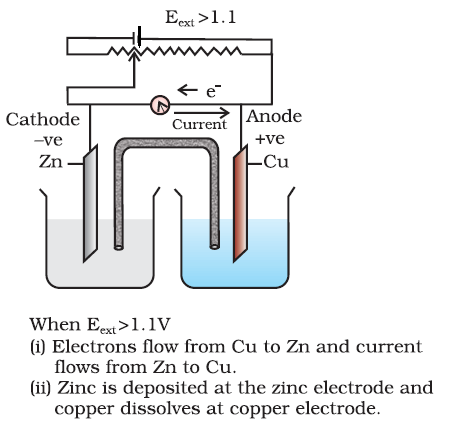Q-4 What is electrode potential ?
A potential difference develops between the electrode and the electrolyte which is called electrode potential.

Q-5 What is standard electrode potential?
When the concentrations of all the species involved in a half-cell is unity (concentration is 1M ) and temperature is 298 K, the electrode potential is known as standard electrode potential.

Q-6 What is cell potential?
The potential difference between the two electrodes of a galvanic cell is called the cell potential and is measured in volts.
What is electrode potential and cell electromotive force (emf)?
The cell potential is the difference between the electrode potentials (reduction potentials) of the cathode and anode. It is called the cell electromotive force (emf) of the cell when no current is drawn through the cell.Under this convention the emf of the cell is positive and is given by the potential of the half-cell on the right hand side minus the potential of the half-cell on the left hand side
i.e.
Ecell = Eright – Eleft

Q-7 How to represent a galvanic cell?
A galvanic cell is generally represented by putting a vertical line between metal and electrolyte solution and putting a double vertical line between the two electrolytes connected by a salt bridge.
For the cell reaction
Cu(s) + 2Ag+(aq) → Cu2+(aq) + 2 Ag(s) The cell can be represented as:
Cu(s)|Cu2+(aq)||Ag+(aq)|Ag(s)

Q-8 Draw the structure and working of standard hydrogen electrode?
The standard hydrogen electrode consists of a platinum electrode coated with platinum black. The electrode is dipped in an acidic solution and pure hydrogen gas is bubbled through it.
H+ (aq) + e →1/2H2(g) The concentration of both the reduced and oxidised forms of hydrogen is maintained at unity (Fig. 3.3). This implies that the pressure of hydrogen gas is one bar and the concentration of hydrogen ion in the solution is one molar.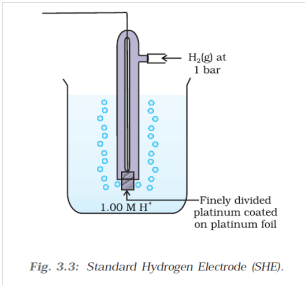Above half-cell called standard hydrogen electrode (Fig.3.3) represented by Pt(s)| H2(g) |H+(aq), is assigned a zero potential at all temperatures corresponding to the reaction.
Q-9 How to measure the standard potential of Cu2+ | Cu
use cupper electrode at right side. Take the concentrations of all the species involved in a half-cell as unity (concentration is 1M )
The measured emf of the cell :
Pt(s) | H2(g, 1 bar) | H+ (aq, 1 M) || Cu2+ (aq, 1 M)| Cu
< style="margin-left:100px;">EӨcell = EӨright – EӨleft Standard hydrogen electrode is at the left
< style="margin-left:100px;">EӨleft =0 plug this values we get
EӨcell = EӨright – 0
EӨcell = EӨright After experiment we get EӨcell = 0.34 V which is the required value

Q- 10 What is the use of platinum or gold in standard hydrogen electrode?
Platinum or gold are used as inert electrodes. They do not participate in the reaction but provide their surface for oxidation or reduction reactions and for the conduction of electrons.

Q-11 What is the Nernst Equation?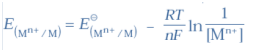R is gas constant (8.314JK–1 mol–1),
F is Faraday constant (96487 C mol–1),
T is temperature in kelvin
[Mn+] is the molar concentration of the species
For Daniell cell, the electrode potential for any given concentration of Cu2+ and Zn2+ ions,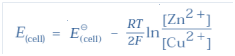At 273 K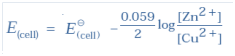Q-12 What is the relation between EӨcell and Kc ?
at equilibrium,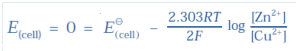For the reaction
Zn(s) + Cu2+(aq) → Zn2+ (aq) + Cu(s)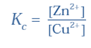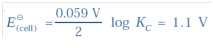After calculation we get
KC = 2 × 1037 at 298K

Q-13 What is the Nernst Equation for the given chemical reaction?
and for a general electrochemical reaction of the type:
a A + bB -(ne-) → cC + dD
Nernst equation can be written as: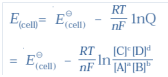Q-14 What is the Gibbs energy of reaction taking place in an electrochemical cell?
Electrical work done in one second is equal to electrical potential multiplied by total charge passed. If we want to obtain maximum work from a galvanic cell then charge has to be passed reversibly. The reversible work done by a galvanic cell is equal to decrease in its Gibbs energy and therefore, if the emf of the cell is E and nF is the amount of charge passed and ΔrG is the Gibbs energy of the reaction, then
Δ rG = – nFE(cell)
It may be remembered that E(cell) is an intensive parameter but ΔrG is an extensive thermodynamic property and the value depends on n. Thus, if we write the reaction
Zn(s) + Cu2+(aq) → Zn2+(aq) + Cu(s)
ΔrG = -2FE(cell)
but when we write the reaction
2 Zn (s) + 2Cu2+(aq) →2 Zn2+(aq)+2Cu(s)
ΔrG = -4FE(cell)
If the concentration of all the reacting species is unity, then E(cell) = (cell) EV and we have
ΔrG_ = –nFEӨ (cell) …(1)
We have the relation
ΔrG_ = –RT ln K. … (2)
From equation (1) and (2)
–nFEӨ (cell) = –RT ln K.
EӨ (cell) = –RT/nF ln K.
Q-15 Conductance of Electrolytic Solutions
The inverse of resistance, R, is called conductance, G, and we have the relation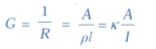The SI unit of conductance is siemens, represented by the symbol ‘S’ and is equal to ohm–1 (also known as mho) or Ω–1. The inverse of resistivity(ρ , rho), called conductivity (specific conductance) is represented by the symbol, κ (Greek, kappa).

Q-16 What is cell constant ?
The quantity l/A is called cell constant denoted by the symbol, G*.It depends on the distance between the electrodes and their area of cross-section and has the dimension of length–1 and can be calculated if we know l and A. Measurement of l and A is not only inconvenient but also unreliable. The cell constant is usually determined by measuring the resistance of the cell containing a solution whose conductivity is already known. For this purpose, we generally use KCl solutions whose conductivity is known accurately at various concentrations and at different temperatures. The cell constant, G*, is then given by the equation:
G* =l/A= Rκ

Q-17 What is a superconductor?
Certain materials called superconductors by definition have zero resistivity or infinite conductivity. Earlier, only metals and their alloys at very low temperatures (0 to 15 K) were known to behave as superconductors,
but nowadays a number of ceramic materials and mixed oxides are also known to show superconductivity at temperatures as high as 150 K.

Q-18 What are the factor effecting conductance of the metal?
The electronic conductance depends on
(i) the nature and structure of the metal
(ii) the number of valence electrons per atom
(iii) temperature (it decreases with increase of temperature).

Q-19 What is ionic conductance and their affecting factors?
The conductivity of electrolytic (ionic) solutions depends on:
(i) the nature of the electrolyte added
(ii) size of the ions produced and their solvation
(iii) the nature of the solvent and its viscosity
(iv) concentration of the electrolyte
(v) temperature (it increases with the increase of temperature).

Q-20 What are Electronically conducting polymers and there advantages?
In 1977 MacDiarmid, Heeger and Shirakawa discovered that acetylene gas can be polymerised to produce a polymer, polyacetylene when exposed to vapours of iodine acquires metallic lustre and conductivity. Since then several organic conducting polymers have been made such as polyaniline, polypyrrole and polythiophene. These organic metals, being composed wholly of elements like carbon, hydrogen and occasionally nitrogen, oxygen or sulphur, are much lighter than normal metals and can be used for making light-weight batteries. Besides, they have the mechanical properties of polymers such as flexibility so that one can make electronic devices such as transistors that can bend like a sheet of plastic. For the discovery of conducting polymers, MacDiarmid, Heeger and Shirakawa were awarded the Nobel Prize in Chemistry for the year 2000.

Q-21 What are the problems takes place in measuring of conductivity of ionic solutions and how they solved ?
1.Passing of direct current (DC) changes the composition of the solution. The first difficulty is resolved by using an alternating current (AC) source

2.A solution cannot be connected to the bridge like a metallic wire or other solid conductor. The second problem is solved by using a specially designed vessel called conductivity cell.

Q-22 What is a conductivity cell?
It is a specially designed vessel which used in measuring of conductivity of ionic solution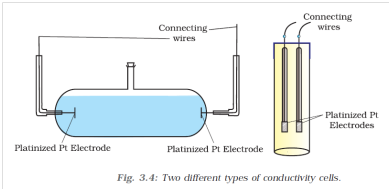Q-23 What is the method to measure conductance using Wheatstone bridge ?
It consists of two resistances R3 and R4, a variable resistance R1 and the conductivity cell having the unknown resistance R2. The Wheatstone bridge is fed by an oscillator O (a source of a.c. power in the audio frequency range 550 to 5000 cycles per second). P is a suitable detector (a headphone or other electronic device) and the bridge is balanced when no current passes through the detector. Under these conditions: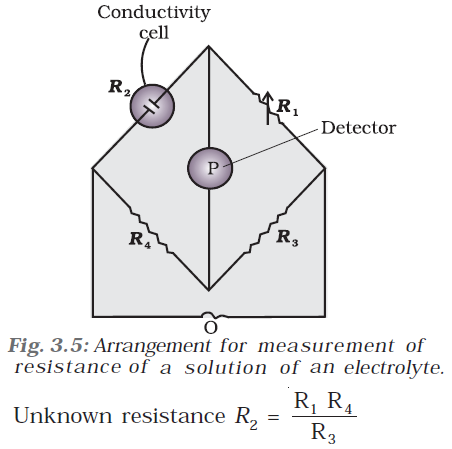Q-24 What is molar conductivity?
Molar conductivity denoted by the symbol Λm (Greek, lambda). Molar conductivity of the solution is equal to conductivity at unity concentration
Mathematically
Molar conductivity,
Λm =c/κ
The unit of κ and concentration is S m–1 and mol m–3 respectively then the units of Λm are in S m2 mol–1.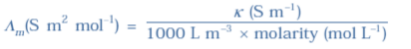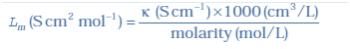Molar conductivity increases with decrease in concentration.
What is limiting Molar conductivity ?
When concentration approaches zero, the molar conductivity is known as limiting molar conductivity and is represented by the symbol Ëm°.
Exaplain the relation between molar conductivity and concentration?
For strong electrolytes, Λ increases slowly with dilution and can be represented by the equation:
Λm = Ëm° – Ac ½
It can be seen that if we plot a Figure. Λm against c1/2, we obtain a straight line with intercept equal to Ëm° and slope equal to ‘–A’. The value of the constant ‘A’ for a given solvent and temperature depends on the type of electrolyte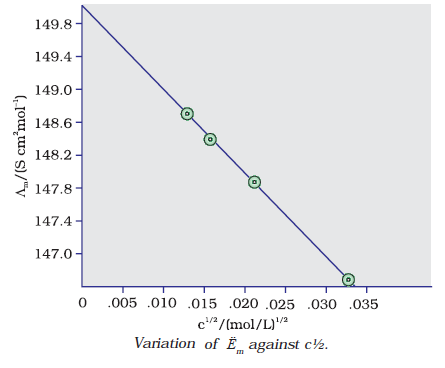i.e., the charges on the cation and anion produced on the dissociation of the electrolyte in the solution. Thus, NaCl, CaCl2, MgSO4 are known as 1-1, 2-1 and 2- 2 electrolytes respectively. All electrolytes of a particular type have the same value for ‘A’.
What is Kohlrausch law of independent migration of ions?
He noted that the difference in Ëm° of the electrolytes NaX and KX for any X is nearly constant .The law states that limiting molar conductivity of an electrolyte can be represented as the sum of the inidual contributions of the anion and cation of the electrolyte. Thus, if λ°Na+ and λ°Cl– are limiting molar conductivity of the sodium and chloride ions respectively, then the limiting molar conductivity for sodium chloride is given by the equation:
Ëm°(NaCl) = λ°Na+ + λ°Cl–
Ëm ° for weak electrolytes is obtained by using Kohlrausch law of independent migration of ions
How to measure equilibrium constant and limiting molar conductivity of week electrolytite?
Weak electrolytes like acetic acid have lower degree of dissociation at higher concentrations and hence for such electrolytes, the change in Λm with dilution is due to increase in the degree of dissociation and consequently the number of ions in total volume of solution that contains 1 mol of electrolyte. In such cases Ëm increases steeply on dilution, especially near lower concentrations. Therefore, Ëm° cannot be obtained by extrapolation of Λm to zero concentration. At infinite dilution (i.e., concentration c → zero) electrolyte dissociates completely (α =1), but at such low concentration the conductivity of the solution is so low that it cannot be measured accurately. Therefore, Ëm ° for weak electrolytes is obtained by using Kohlrausch law of independent migration of ions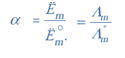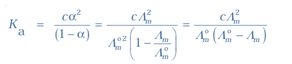What are the Faraday’s Laws of Electrolysis?
First law
The amount of chemical reaction which occurs at any electrode during electrolysis by a current is proportional to the quantity of electricity passed through the electrolyte (solution or melt).
Second law
The amounts of different substances liberated by the same quantity of electricity passing through the electrolytic solution are proportional to their chemical equivalent weights (Atomic Mass of Metal ÷ Number of electrons required to reduce the cation).
It is obvious that one mole of Mg2+ and Al3+ require 2 mol of electrons (2F) and 3 mol of electrons (3F) respectively. 1 F = 96487 C mol–1
Products of Electrolysis
The products of electrolysis depend on the different oxidizing and reducing species present in the electrolytic cell and their standard electrode potentials. some of the electrochemical processes although feasible, are so slow kinetically that at lower voltages these don’t seem to take place and extra potential (called overpotential) has to be applied, which makes such process more difficult to occur.
For example on electrolysis of water at anode
At higher potential we get
Cl (aq) → ½ Cl2 (g) + e– E(cell) V= 1.36 V
At low potential
2H2O(l )→ O2(g) + 4H+(aq) + 4e E(cell)V = +1.23 V,
Explain type of cells ?
In the primary batteries, the reaction occurs only once and after use over a period of time battery becomes dead and cannot be reused again.
Example
< class="fontx" align="left">< style="float:left; "> Leclanche cell : The cell consists of a zinc container that also acts as anode and the cathode is a carbon (graphite) rod surrounded by powdered manganese dioxide and carbon (Fig.3.8). The space between the electrodes is filled by a moist paste of ammonium chloride (NH4Cl) and zinc chloride (ZnCl2).
Anode: Zn(s) → Zn2+ + 2e-
Cathode: MnO2+ NH4+ + e- → MnO(OH) + NH3
The cell has a potential of nearly 1.5 V.
Use : write your self < style="float:right">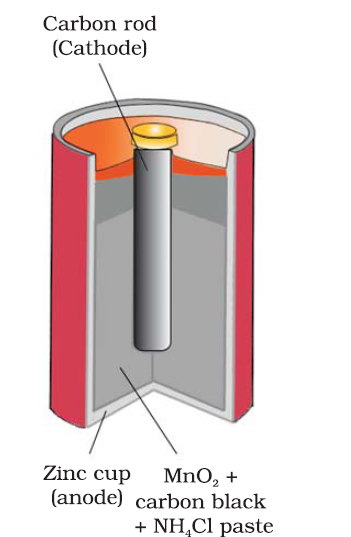< class="fontx" align="left">< style="float:left; width:auto">Mercury cell
consists of zinc – mercury amalgam as anode and a paste of HgO and carbon as the cathode. The electrolyte is a paste of KOH and ZnO. The electrode reactions for the cell are given below:
Anode: Zn(Hg) + 2OH- → ZnO(s) + H2O + 2e-
Cathode: HgO + H2O + 2e- → Hg(l ) + 2OH-
The overall reaction is represented by
Zn(Hg) + HgO(s) → ZnO(s) + Hg(l )
The cell potential is approximately 1.35 V and remains constant during its life as the overall
< style="float:right;">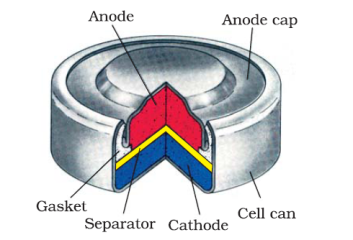Secondary Batteries
A secondary cell after use can be recharged by passing current through it in the opposite direction so that it can be used again.
Example
< class="fontx" align="left">< style="float:left;">Lead storage battery
It consists of a lead anode and a grid of lead packed with lead dioxide (PbO2 ) as cathode. A 38% solution of sulphuric acid is used as an electrolyte. The cell reactions when the battery is in use are given below:
Anode: Pb(s) + SO42–(aq) → PbSO4(s) + 2e
Cathode: PbO2(s) + SO42–(aq) + 4H+(aq) + 2e → PbSO4 (s) + 2H2O (l )
i.e., overall cell reaction consisting of cathode and anode reactions is:
Pb(s)+PbO2(s)+2H2SO4(aq)→ 2PbSO4(s) + 2H2O(l)
On charging the battery the reaction is reversed and PbSO4(s) on
anode and cathode is converted into Pb and PbO2, respectively.

< style="float:right;">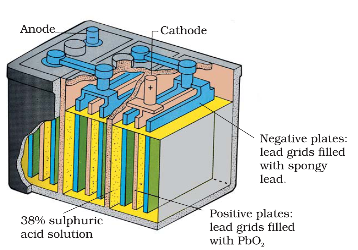< class="fontx" align="left"> Nickel cadmium cell
longer life than the lead storage cell but more expensive to manufactureoverall reaction during discharge is:
Cd (s)+2Ni(OH)3 (s) → CdO (s) +2Ni(OH)2 (s) +H2O(l )
Write mechanism or advantages of hydrogen cell?
In fuel Cellshydrogen and oxygen are bubbled through porous carbon electrodes into concentrated aqueous sodium hydroxide solution. Catalysts like finely ided platinum or palladium metal are incorporated into the electrodes for increasing the rate of electrode reactions. The electrode reactions are given below :
Cathode: O2(g) + 2H2O(l ) + 4e- → 4OH-(aq)
Anode: 2H2 (g) + 4OH-(aq) → 4H2O(l) + 4e-
Overall reaction being:
2H2(g) + O2(g) → 2 H2O(l )
(1) The cell runs continuously as long as the reactants are supplied.
(2) Fuel cells produce electricity with an efficiency of about 70 % compared to thermal plants whose efficiency is about 40%.
(3) Fuel cells are pollution free and in view of their future importance
(4) The water vapours produced during the reaction were condensed and added to the drinking water supply

What is corrosion explain how corrosion works as a cell ?
Corrosion
Corrosion slowly coats the surfaces of metallic objects with oxides or other salts of the metal. The rusting of iron, tarnishing of silver, development of green coating on copper and bronze are some of the examples of corrosion.
Rusting:
At a particular spot (Fig. 3.13) of an object made of iron, oxidation takes place and that spot behaves as anode and we can write the reaction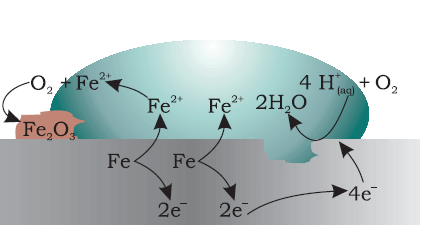Anode: 2 Fe (s)→2 Fe2+ + 4 eE(Fe2+/Fe)V= – 0.44 V
Electrons released at anodic spot move through the metal and go to another spot on the metal and reduce oxygen in presence of H+
Cathode: O2(g) + 4 H+(aq) + 4 e→2 H2O (l )E=1.23 V
The overall reaction being:
2Fe(s)+O2(g) + 4H+(aq)→2Fe2+(aq)+ 2 H2O (l )E(cell)V=1.67 V

What is hydrogen economy?
At present the main source of energy that is driving our economy is fossil fuels such as coal, oil and gas. As more people on the planet aspire to improve their standard of living, their energy requirement will increase. In fact, the per capita consumption of energy used is a measure of development. Of course, it is assumed that energy is used for productive purpose and not merely wasted. We are already aware that carbon dioxide produced by the combustion of fossil fuels is resulting in the ‘Greenhouse Effect’. This is leading to a rise in the temperature of the Earth’s surface, causing polar ice to melt and ocean levels to rise. This will flood low-lying areas along the coast and some island nations such as Males face total submergence. In order to avoid such a catastrope, we need to limit our use of carbonaceous fuels. Hydrogen provides an ideal alternative as its combustion results in water only. Hydrogen production must come from splitting water using solar energy. Therefore, hydrogen can be used as a renewable and non polluting source of energy. This is the vision of the Hydrogen Economy. Both the production of hydrogen by electrolysis of water and hydrogen combustion in a fuel cell will be important in the future. And both these technologies are based on electrochemical principles.

## Exercise Chapter 3 Class 12 Chemistry ELECTROCHEMISTRY Ncert Solutions

Question 1:Arrange the following metals in the order in which they displace each other from the solution of their salts.
Al, Cu, Fe, Mg and Zn

Answer:A metal is able to displace other metal from their salt solution if the former metal has lower electrode potential
We get the following order when put the metal in order of their increasing electrode potential
Mg(–2.37), Al(–1.66) , Zn (–0.76), Fe(–0.44), Cu(0.34)

Question 2:Given the standard electrode potentials,
K+/K = −2.93V, Ag+/Ag = 0.80V,
Hg2+/Hg = 0.79V
Mg2+/Mg = −2.37 V, Cr3+/Cr = − 0.74V Arrange these metals in their increasing order of reducing power.

Answer:Reducing power of metals increase with decrease of reduction potential. Hence the increasing order of reducing power will
Ag < Hg < Cr < Mg < K

Question 3:Depict the galvanic cell in which the reaction Zn(s) + 2Ag+(aq) → Zn2+(aq) + 2Ag(s)
takes place. Further show:
(i) Which of the electrode is negatively charged?
(ii) The carriers of the current in the cell.
(iii) Inidual reaction at each electrode.

Answer:The galvanic cell in which the given reaction takes place is depicted as:
(i) Zn electrode act ads anode in galvanic cell so it will be negatively charged.
(ii) Electrons move anode to cathode in the external circuit, and the direction of flow of current is always opposite to the flow of electron so that current will flow from silver(cathode) to zinc(anode).
(iii)
At anode
Zn(s) ––– > Zn2+(aq) + 2e
At cathode
Ag+ + e ––– > Ag(s)

Question 4:Calculate the standard cell potentials of galvanic cells in which the following reactions
take place:
(i) 2Cr(s) + 3Cd2+(aq) → 2Cr3+(aq) + 3Cd
(ii) Fe2+(aq) + Ag+(aq) → Fe3+(aq) + Ag(s)
Calculate the =∆rGθ and equilibrium constant of the reactions.

The galvanic cell of the given reaction is represented as
Cr(s) | Cr3+ || Cd2+ | Cd(s)
The formula of standard cell potential is
E°cell = E° right – E°left
E°cell = E° Cd – R°Cr
E°cell = – 0.40 –( – 0.74 )
E°cell = – 0.40 –( – 0.74 )
E°cell = + 0.43 V
In balanced reaction there are 6 electron are transferring so that n = 6
Faraday constant, F = 96500 C mol−1
E°cell = + 0.34 V
Use formula
∆rGθ = – nFE°cell
Plug the value we get
Then, = −6 × 96500 C mol−1 × 0.34 V
= −196860 CV mol−1
= −196860J mol−1
= −196.86 kJ mol−1
Again,
Use second formula of ∆rGθ
∆rGθ = −2.303RT log kC
log KC = (∆rGθ) /( – 2.303RT)
plug the values we get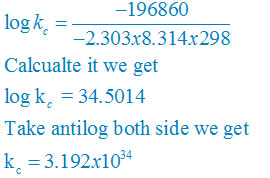(ii)
The galvanic cell of the given reaction is represented as
Fe2+(aq) | Fe3+(aq) || Ag+ | Ag(s)
The formula of standard cell potential is
E°cell = E° right – E°left
E°cell = 0.80 – 0.77
E°cell = + 0.03 V
In balanced reaction there are 1 electron are transferring so that n = 1
Faraday constant, F = 96500 C mol−1
E°cell = + 0.03 V
Use formula
∆rGθ = – nFE°cell
Plug the value we get
Then, = −1 × 96500 C mol−1 × 0.03 V
= −2895 CV mol−1
= −2895J mol−1
= −2.895 kJ mol−1
Again,
Use second formula of ∆rGθ
∆rGθ = −2.303RT log kC
log KC = (∆rGθ) /( – 2.303RT)
plug the values we get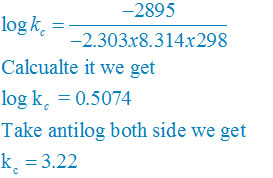Question 5:Write the Nernst equation and emf of the following cells at 298 K:
(i) Mg(s) | Mg2+(0.001M) || Cu2+(0.0001 M) | Cu(s)
(ii) Fe(s) | Fe2+(0.001M) || H+(1M)|H2(g)(1bar) | Pt(s)
(iii) Sn(s) | Sn2+(0.050 M) || H+(0.020 M) | H2(g) (1 bar) | Pt(s)
(iv) Pt(s) | Br2(l) | Br(0.010 M) || H+(0.030 M) | H2(g) (1 bar) | Pt(s).

(i)Net reaction
Mg(s) +Cu2+(aq)↔ Mg2+(aq) + Cu(s)
Nernst equation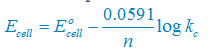There are two electron are transferring so that n = 2
E°cell = E° right – E°left
E°cell =+0.34 – (–2.37)V
E°cell =+2.71V
Plug the value we get
Concentration of solid substance is 1 always so that [Mg] = [Cu] =1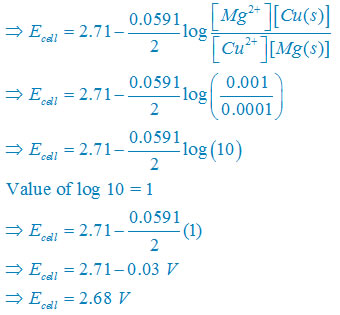(ii) Net reaction
Fe(s) +2H+(aq)↔ Fe2+(aq) + H2(g)
Nernst equationThere are two electron are transferring so that n = 2
E°cell = E° right – E°left
E°cell =0– (–0.44)V
E°cell =+0.44 V
Plug the value we get
Concentration of solid substance is 1 always so that [Fe] = [H2] =1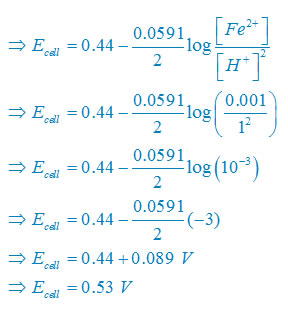(iii) Net reaction
Sn(s) +2H+(aq)↔ Sn2+(aq) + H2(g)
Nernst equationThere are two electron are transferring so that n = 2
E°cell = E° right – E°left
E°cell =0– (–0.14)V
E°cell =+0.14 V
Plug the value we get
Concentration of solid substance is 1 always so that [Sn] = [H2] =1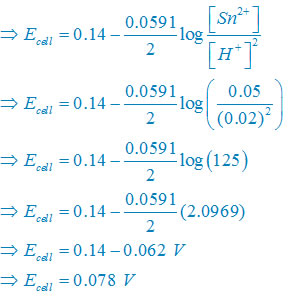(iv) Net reaction
2Br(aq) + 2H+(aq)↔ Br2(l) + H2(g)
Nernst equationThere are two electron are transferring so that n = 2
E°cell = E° right – E°left
E°cell =0– (1.08)V
E°cell –1.08V
Plug the value we get
Concentration of solid substance is 1 always so that [Br2] = [H2] =1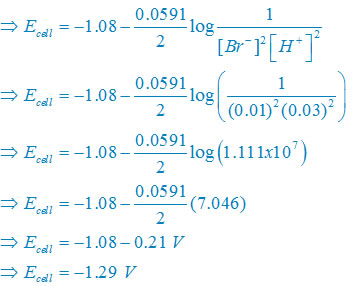Question 6:In the button cells widely used in watches and other devices the following reaction takesplace:
Zn(s) + Ag2O(s) + H2O(l) → Zn2+(aq) + 2Ag(s) + 2OH(aq)
Determine and for the reaction.

The formula of standard cell potential is
E°cell = E° right – E°left
Use this link to get all values
http://ncerthelp.blogspot.in/2013/04/the-standard-electrode-potentials-at.html
E°cell = 0.344 – ( – 0.76)
E°cell = 0.344+0.076 V
E°cell = +1.104 V

In balanced reaction there are 2 electron are transferring so that n = 2
Faraday constant, F = 96500 C mol−1
E°cell = + 1.104 V
Use formula
∆rGθ = – nFE°cell
Plug the value we get
Then, = −2 × 96500 C mol−1 × 1.104 V
= −212304 CV mol−1
= −212304J mol−1
= −212.304kJ mol−1
= −213.04 kJ

Question 7:Define conductivity and molar conductivity for the solution of an electrolyte. Discuss their variation with concentration.

Conductivity :–
Conductivity of a solution is equal to the conductance of a solution of 1 cm length and cross section area of 1 square cm. it may also be define as the conductance of ine centimeter cube of the conductor . It is represented by the symbol Kappa (κ). mathematically we can write
κ = 1/ p
here ρ is resistivity
the unit of K is ohm –1 cm –1 or S cm–1
The conductivity, κ, of an electrolytic solution depends on the concentration of the electrolyte, nature of solvent and temperature.

Molar conductivity:
Molar conductivity of a solution at a given concentration is the conductance of the volume V of solution containing one mole of electrolyte kept between two electrodes with area of cross section A and distance of unit length. Therefore,
Distance is unit so l = 1
Volume = area of base × length
So V = A × 1 = A
Λm =κA/l
Λm = κV
Or
Molar conductivity increases with decrease in concentration. When concentration approaches zero, the molar conductivity is known as limiting molar conductivity and is represented by the symbol Ë°m.
For strong electrolytes, Λ increases slowly with dilution and can be represented by the equation:
Λm = Ë°m° – A c ½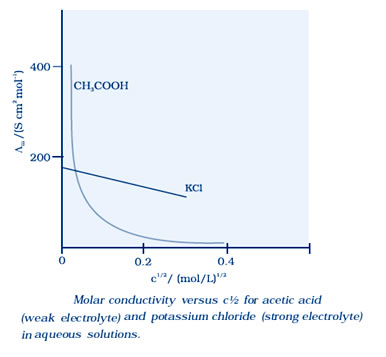It can be seen that if we plot Λm against c1/2, we obtain a straight line with intercept equal to Ëm° and slope equal to ‘–A’. The value of the constant ‘A’ for a given solvent and temperature depends on the type of electrolyte i.e., the charges on the cation and anion produced on the dissociation of the electrolyte in solution.

Question 8:The conductivity of 0.20 M solution of KCl at 298 K is 0.0248 Scm−1. Calculate its molar conductivity.

κ = 0.0248 S cm−1 and c = 0.20 M
Formula of molar conductivity,
Λm = ( k × 1000)/M
Λm =(0.0248 × 1000 )/0.2
Λm =124 S cm2 mol−1

Question 9:The resistance of a conductivity cell containing 0.001M KCl solution at 298 K is 500M. What is the cell constant if conductivity of 0.001M KCl solution at 298 K is 0.146 × 10−3S cm−1.

Conductivity of the cell , κ = 0.146 × 10−3 S cm−1
Resistance of the cell , R = 1500 Ω
Formula of cell constant
Cell constant = κ × R
Plug the values we get
= 0.146 × 10−3 × 1500
= 0.219 cm−1

Question 10:The conductivity of sodium chloride at 298 K has been determined at different concentrations and the results are given below: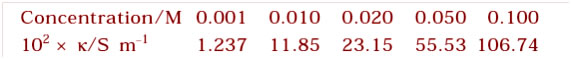Calculate Λm for all concentrations and draw a plot between Λm and c½. Find the value of Λ°m .

Divide by 102 to get k/sm–1
Divide by 100 to concert m–1 to cm–1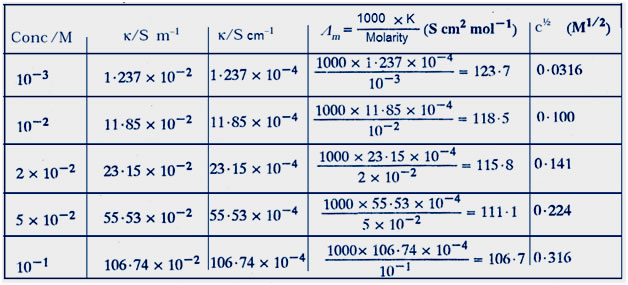Now draw the graph between Λm and c½.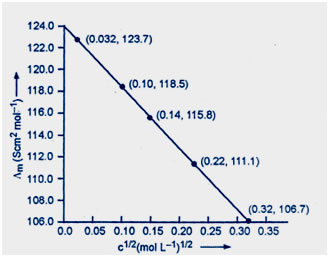At zero concentration value of Λm = 124 so that the value of Λ°m = 124.0 S cm2 mol–1

Question 11: Conductivity of 0.00241 M acetic acid is 7.896 × 10−5 S cm−1. Calculate its molar conductivity and if for acetic acid is 390.5 S cm2 mol−1, what is its dissociation constant?

Answer:Given that , κ = 7.896 × 10−5 S m−1
C=M= 0.00241 mol L−1
The formula of molar conductivity,
Λm = (k × 1000)/M
Plug the value we get
Λm = (7.896 × 10−5 × 1000)/ 0.00241
= 32.76S cm2 mol−1
The formula of degree of dissociation
α = Λm/ Λ°m
Plug the value we get
α = 32.76S/390.5
= 0.084
The formula of dissociation constant
K = Cα/(1 – α)
Plug the values we get
K = 0.00241 × 0.084/(1– 0.084)
= 1.86 × 10−5 mol L−1

Question 12:How much charge is required for the following reductions:
(i) 1 mol of Al3+ to Al.
(ii) 1 mol of Cu2+ to Cu.
(iii) 1 mol of MnO4– to Mn2+.

Formula required charge n × F
n = difference of charge on ions
F is constant and equal to 96487 Coulombs
Here n = 3
Hence required charge = 3 × 96487 Coulombs
= 289461 Coulombs
= 2.89 ×10 –5 Coulombs
(ii)

n = 2
plug the value in formula we get
Required charge= 2 × 96487 Coulombs
= 192974 Coulombs
= 1.93 × 105 Coulombs
(iii)
Charge on Mn in MnO4–
Charge on Oxygen is – 2
Mn + 4O = – 1
Mn +4(–2) = – 1
Mn = +7
So our reaction is
MN7+ → Mn2+
n = 7– 2 = 5
Required charge will = 5 × 96487 Coulombs
= 482435 Coulombs
= 4.82 × 105 Coulombs

Question 13:How much electricity in terms of Faraday is required to produce
(i) 20.0 g of Ca from molten CaCl2.
(ii) 40.0 g of Al from molten Al2O3.

The balance reaction will
The charge on Ca in CaCl2
Ca + 2Cl = 0
Cl has –1 charge so that
Ca + 2(–1) = 0
Ca = 2
We have to get 2o g Ca from Ca2+
Number of required moles = mass / molar mass
Molar mass of Ca is 40 g/mol and required mass of Ca is 20 g
Hence number of moles = 20/40 = 0.5 mol
Electricity required to produce 1 mol of calcium = 2 F
The electricity required to produce 0.5 mol of calcium
= 0.5 × 2 F
= 1 F
(ii)
Charge on Al in Al2O3
2Al + 3O = 0
Oxygen has –2
2Al +3(–2) = 0
Al = 3
Change transfer n = 3
Charge required for 1 mol of Al = 3F
Number of moles of Al = 40 /27 = 1.48

The electricity required to produce 1.48 mol of Al = 1.48 × 3 F
= 4.44 F

Question 14: How much electricity is required in coulomb for the oxidation of (i) 1 mol of H2O to O2. (ii) 1 mol of FeO to Fe2O3.

Answer:(i) We have 1 mol of H2O
1 mol of H2O will give one atom of O
Charge on O = – 2
Electricity required =n F
= 2 × 96487 Coulombs
= 192974 Coulombs
= 1.93 × 105 Coulombs

(ii)
Charge on Fe in compound FeO = + 2
Charge on Fe in compound Fe2O3 = + 3
Change in charge n = 1
Electricity required = nF
= 96487 Coulombs
= 9.65 × 104 Coulombs

Question 15: A solution of Ni(NO3)2 is electrolysed between platinum electrodes using a current of 5 amperes for 20 minutes. What mass of Ni is deposited at the cathode?

Current, I = 5A
Time, t = 20 × 60 = 1200 s (1 min = 60 second)
Charge = I × t
= 5 × 1200
= 6000 Coulombs
Charge on Ni
NO3 has –1 charge always so that
Ni +2(NO3) =0
Ni + 2(–1) = 0
Ni = +2
Charge required to deposited 1 mol of Ni = nF
= 2 × 96487 Coulombs
= 192974 Coulombs
1 mol of Ni = 58.7 g (use periodic table to get this value)
192974 Coulombs will generate = 58.7 g of Ni
6000 Coulombs will generate = 58.7 g × 6000 Coulombs /192974 Coulombs
= 1.825 g

Hence, 1.825 g of nickel will be deposited at the cathode.

Question 16:Three electrolytic cells A,B,C containing solutions of ZnSO4, AgNO3 and CuSO4, respectively are connected in series. A steady current of 1.5 amperes was passed through them until 1.45 g of silver deposited at the cathode of cell B. How long did the current flow? What mass of copper and zinc were deposited?

Answer:Ag has +1 charge in AgNO3
So charge transfer, n = 1
F = 96487 Coulombs
Molar mass of Ag = 108 g
Use formula required charge = nF
So required charge for 1 mol or 108 g of Ag = Coulombs.
Charge required for 1.45 g of Ag = 96487 Coulombs × 1.45g/108g
= 1295.43 Coulombs
Given that current I = 1.5 A
Use formula Charge = I × t
Time t = charge / I
= 1295.43 Coulombs/ 1.5 A
= 863.6 s
Divide by 60 to convert in minute
= 864/60 min
= 14.40 min
Charge in Cu in CuSo4 is +2
Use formula required charge for 1 mol = nF
So charge required for 1 mol or 63.5 g of Cu = 2 × 96487 = 192974 Coulombs
192974 Coulombs of charge deposit = 63.5 g of Cu
1295.43 Coulombs of charge will deposit = 63.5 g × 1295.43/192974
= 0.426 g of Cu
Similarly for Zn
Zn has charge in ZnSO4 = +2
Use formula required charge for 1 mol = nF
So charge required for 1 mol or 63.5 g of Zn = 2 × 96487 = 192974 Coulombs
192974 Coulombs of charge deposit = 63.5 g of Zn
2 × 96487 C of charge deposit = 65.4 g of Zn
1295.43 Coulombs of charge will deposit = 65.4 g × 1295.43/192974
= 0.439 g of Zn

Question 17: Using the standard electrode potentials given in Table 3.1, predict if the reaction between the following is feasible:
(i) Fe3+(aq) and I(aq)
(ii) Ag+ (aq) and Cu(s)
(iii) Fe3+ (aq) and Br(aq)
(iv) Ag(s) and Fe3+(aq)
(v) Br2 (aq) and Fe2+ (aq).

∆rGθ < 0
And ∆rGθ = – nFE°cell so that
– nFE°cell < 0
n and F both are always positive values
so that
–E°cell < 0
Change the sign we get
E°cell > 0
Hence for any feasible reaction E°cell will always positive
(i)
Use this link to get all values of E°
http://ncerthelp.blogspot.in/2013/04/the-standard-electrode-potentials-at.html
Balance possible half reactions are
2Fe3+ + 2e- →2Fe+2 E° = +0.77 V
2I → I2 + 2e- E° = –0.54 V
E°cell = +0.23
Here E°cell > 0 so reaction is possible
(ii)
2Ag+ + 2e- →2Ag E° = +0.80 V
Cu → Cu+2 + 2e- E° = –0.34 V
E°cell = +0.46V
Here E°cell > 0 so reaction is possible
(iii)
2Fe3+ + 2e- →2Fe+2 E° = +0.77 V
2Br → Br2 + 2e- E° = –1.09 V
E°cell = –0.32V
Here E°cell < 0 so reaction is not possible
(iv)
2Ag →2Ag+1 + 2e- E° = – 0.80 V
2Fe3+ + 2e- →2Fe+2 E° = +0.77 V
E°cell = –0.03V
Here E°cell < 0 so reaction is not possible
(V)
Br2 + 2e- →2Br E° = +1.09 V
2Fe2+ → 2Fe3+ + 2e- E° = – 0.77 V
E°cell = +0.32V
Here E°cell > 0 so reaction is possible

Question 18: Predict the products of electrolysis in each of the following:
(i) An aqueous solution of AgNO3 with silver electrodes.
(ii) An aqueous solution of AgNO3with platinum electrodes.
(iii) A dilute solution of H2SO4with platinum electrodes.
(iv) An aqueous solution of CuCl2 with platinum electrodes.

All ions are in aqueous state
(i)
Reaction in solution
AgNO3 ↔ Ag+ + NO3–
H2O ↔ H+ + OH
Reaction at cathode
Ag+ + e-→ Ag
Reaction at anode
Ag(s) + NO3–→ AgNO3(aq) + e-
Hence Ag will deposit at cathode and dissolve at anode
(ii)
Reaction in solution
AgNO3 ↔ Ag+ + NO3–
H2O ↔ H+ + OH
Reaction at cathode
Ag+ + e-→ Ag
Reaction at anode
Due to platinum electrode self of ionization of water will take place
H2O → 2H+ + 1/2O2(g) + 2e-
Hence Ag will deposit at cathode and O2 gas will generate at anode
(iii)
Reaction in solution
H2SO4 ↔ 2H+ + SO42–
H2O ↔ H+ + OH
Reaction at cathode
H+ + e-→ ½ H2
Reaction at anode
Due to platinum electrode self of ionization of water will take place
H2O → 2H+ + 1/2O2(g) + 2e-
Hence H2 gas will generate at cathode and O2 gas will generate at anode
(iv)
Reaction in solution
CuCl2(s) ↔ Cu2+ + 2Cl
H2O ↔ H+ + OH
Reaction at cathode
Cu2+ + 2e-→ Cu(S)
Reaction at anode
2Cl → Cl2 + 2e-
Hence Cu will deposit at cathode and Cl2 gas will generate at anode

## In text solution Chapter 3 Class 12 Chemistry ELECTROCHEMISTRY Ncert Solutions

Question 3.1: How would you determine the standard electrode potential of the systemMg2+ | Mg?

Answer:Use standard hydrogen electrode as anode and Mg2+ | Mg as a cathode we can measure the standard electrode potential of systemMg2+ | Mg. Standard hydrogen electrode, represented by Pt(s), H2(g) (1 atm) | H+ (aq) and dip the electrode of Magnesium wire in a 1M MgSO4 solution .The standard hydrogen electrode is always zero.
Use formula
E°cell = E° right – E°left
The standard hydrogen electrode is always zero.
So that the value of
E°left =0
Hence
E°cell = E° Mg|Mg2+
Or
E° Mg|Mg2+= E°cell

Question 3.2: Can you store copper sulphate solutions in a zinc pot?

Answer:In reactivity table zink is at head of cupper so that zinc is more reactive than copper. So that , zinc can displace copper from its salt solution. Hence the solution of copper sulphate cannot be stored in a zinc pot.

Question 3.3:Consult the table of standard electrode potentials and suggest three substances that an oxidise ferrous ions under suitable conditions.

Answer:Any substance which standard electrode potential is more than that of Fe3+ /F2+ can oxidise ferrous ions.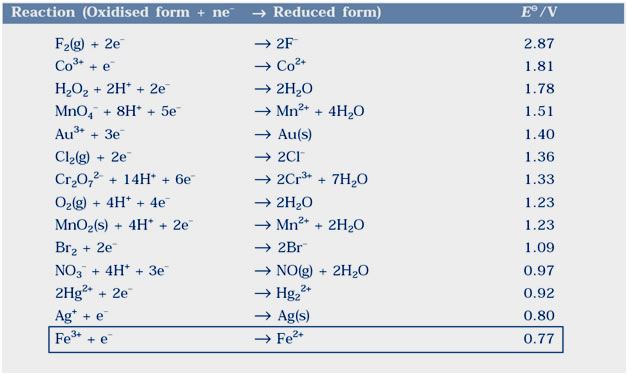All the elements (like F2,Cl2 and O2) of the table can oxidized ferrous ions

Question 3.4:Calculate the potential of hydrogen electrode in contact with a solution whose pH is 10.

given that pH = 10
use formula [H+] = 10pH
so that [H+] = 10−10 M
Electrode reaction will
H+ + e →1/2 H2
Use the formula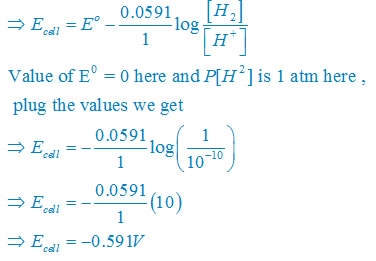Question 3.5: Calculate the emf of the cell in which the following reaction takes place: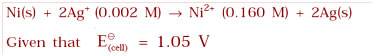[Ag+] = 0.002 M
[Ni2+] =0.160 M
n = 2
Apply the Nernst equation: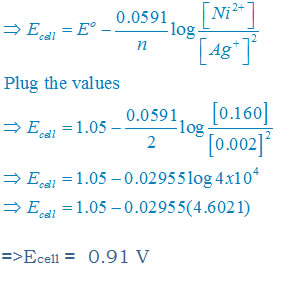Question 3.6: The cell in which the following reactions occurs: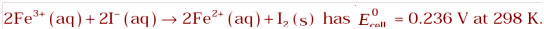Calculate the standard Gibbs energy and the equilibrium constant of the cell reaction.

n = 2
T = 298 K
E°cell = 0.236 V
We have the formula
∆rGθ = – nFE°cell
Plug the values, we get
∆rGθ = −2 × 96487 × 0.236
∆rGθ = −45541.86 J mol−1
Divide by 100o to convert in KJ
∆rGθ = −45.54 kJ mol−1
Use second formula of ∆rGθ
∆rGθ =−2.303RT log Kc
Plug the values we get
−45541.86 J mol−1 = –2.303 × 8.314 × 298 log Kc
Solve it we get
Log Kc = 7.98
Take antilog both side, we get
Kc = Antilog (7.98)
= 9.6 × 107

Question 3.7: Why does the conductivity of a solution decrease with dilution?

Answer:The conductivity of a solution is directly proportional to number of ions present in a unit volume of the solution because current is carried forward by the ions. With dilution number of ions in unit volume decreases so that conductivity also decreases. Hence with dilution conductivity decreases

Question 3.8:Suggest a way to determine the Λ°m value of water.

Λ°m(H2O) = Λ°m(HCl) + Λ°m(NaOH) – Λ°m(NaCl)
Hence if we know the values of Λ°m for HCl, NaOH, and NaCl, then we can calculate the value of Λ°m for the water

Question 3.9:The molar conductivity of 0.025 mol L−1 methanoic acid is 46.1 S cm2 mol−1. Calculate its degree of dissociation and dissociation constant. Given λ0(H+)= 349.6 S cm2 mol–1 and λ0(HCOO) = 54.6 S cm2 mol–1

λ0(H+)= 349.6 S cm2 mol–1
λ0(HCOO) = 54.6 S cm2 mol–1
Concentration ,C = 0.025 mol L-1
λ(HCOOH) = 46.1 S cm2 mol−1
use formula
λ°(HCOOH)= λ0(H+) + λ0(HCOO)
plug the values we get
λ°(HCOOH)= 0.349.6 + 54.6
=404.2 S cm2 mol−1
Formula of degree of dissociation:
ά = λ°(HCOOH)/ λ°(HCOOH)
ά = 46.1 / 404.2
ά = 0.114
Formula of dissociation constant:
K = (c ά2)/(1 – ά)
Plug the values we get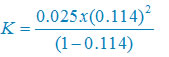K = 3.67 × 10–4 mol per liter

Question 3.10:If a current of 0.5 ampere flows through a metallic wire for 2 hours, then how many electrons would flow through the wire?

Time, t = 2 hours
Convert in sec we get
Time, t = 2 × 60 × 60 s
= 7200 s
Use formula
Charge Q = It
Plug the values we get
= 0.5 A × 7200 s
= 3600 Coulombs
Number of electrons = total charge / charge on 1 electrons
= 3600/(1.6 ×1019)
=2.25 × 10M22 electrons

Question 3.11:Suggest a list of metals that are extracted electrolytically.

Answer:The Metals which is at the top of the reactivity series can extracted electrolytically.
So all alkali metals and some alkaline metals can extracted with this method
Example Li, Na, K, Mg, Al etc.

Question 3.12:Consider the reaction:
Cr2O72– + 14H+ + 6e → 2Cr3+ + 8H2O
What is the quantity of electricity in coulombs needed to reduce 1 mol of Cr2O72– ?

Answer:In given equation there are 6 electrons are required so that n = 6
Use the formula
Required charge = nF
Plug the values in this formula we get
Required charge = 6 × 96487 Coulombs
= 578922 Coulombs

= 5.79 × 105 Coulombs

Question 3.14:Suggest two materials other than hydrogen that can be used as fuels in fuel cells.

(1)Methane
(2)Methanol

Question 3.15: Explain how rusting of iron is envisaged as setting up of an electrochemical cell.

Answer:In process of rusting, water molecules present at the layer of iron react with oxides and get dissociated and give H+ ions
H2O + CO2 → H2CO3
H2CO3 ↔ 2H+ + CO32–
In present of H+ ions iron convert in Fe2+ so this part is act as anode
Reaction at anode:
Fe(s) → Fe2+(aq) + 2e
Electron released at anodic spot move through the metals and go to another spot on the metal and reduce oxygen this spot act as cathode
Reaction at Cathode:
O2(g)+ 4H+(aq) + 4e+( aq) → 2H2O(l)

The overall reaction
2Fe(s) + O2(g) + 4H+(aq) → 2H2O(l) + Fe2+(aq)

Hence, rusting of iron act as electrochemical cell.

### NCERT Books Free Pdf Download for Class 5, 6, 7, 8, 9, 10 , 11, 12 Hindi and English Medium

 Mathematics Biology Psychology Chemistry English Economics Sociology Hindi Business Studies Geography Science Political Science Statistics Physics Accountancy